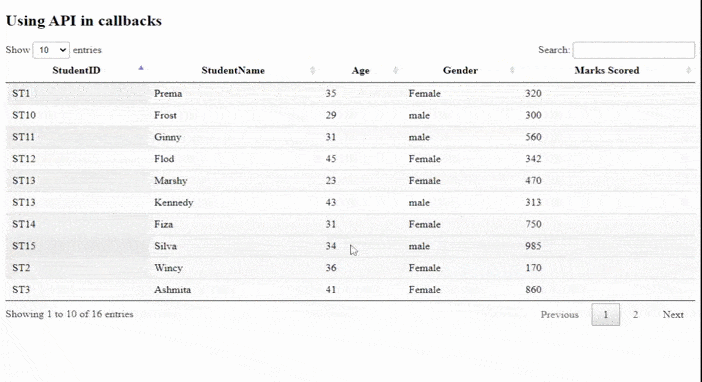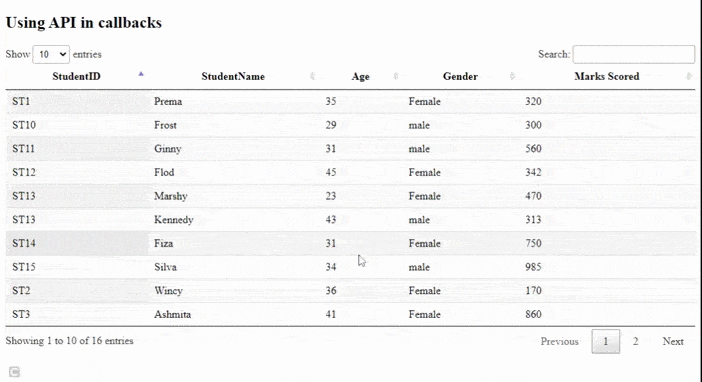GeeksforGeeks App
Open AppBrowser
Continue

# How to use API inside callbacks using jQuery DataTables plugin ?

Datatables are a modern jQuery plugin for adding interactive and advanced controls to HTML tables for our webpages. DataTables is a simple-to-use jQuery plug-in with many options available for developer’s custom changes. The other common features are pagination, searching, sorting, and multiple column ordering.

In this article, we will learn to use API inside callbacks. A very simple example is shown, the developers can add on functionalities as per the application’s need.

The pre-compiled files which are needed to implement are:

`https://cdn.datatables.net/1.10.22/css/jquery.dataTables.min.css`

`//cdn.datatables.net/1.10.22/js/jquery.dataTables.min.js`

Approach: All the above files are included for further implementation which is used for handling datatables. A simple student table is used with their details like student id, name, age, gender, and marks scored. The table is initialized using the jQuery DataTable plugin in the JavaScript part of the code. On click event, it gets the cell nodes and draws the result. The plugin’s \$().API method is used to get all cell nodes in the table’s body and then the relevant action is taken on them. A filter is applied to the table with the value inside the cell.

Example: The following example shows the use of the API inside the click event callbacks. The Initcomplete ( initialization complete callback) is useful to know when the student table is initialized, fully loaded, and drawn. It’s useful to know for configuring components connected to table.

## HTML

 ```<``html``>``  ``<``head``>``    ````    ``<``link``      ``rel``=``"stylesheet"``      ``href``=``"https://cdn.datatables.net/1.10.22/css/jquery.dataTables.min.css"``/>``    ````    ``<``script``      ``type``=``"text/javascript"``      ``src``=``"https://code.jquery.com/jquery-3.5.1.js"``>``    ````    ````    ``<``script``      ``type``=``"text/javascript"``      ``src``=``"https://cdn.datatables.net/1.10.22/js/jquery.dataTables.min.js"``>``    ````  ```` ` `  ``<``body``>``    ``<``h2``>Using API in callbacks``    ````    ``<``table` `id``=``"tableID"` `class``=``"display"` `           ``style``=``"width: 100%"``>``      ``<``thead``>``        ``<``tr``>``          ``<``th``>StudentID``          ``<``th``>StudentName``          ``<``th``>Age``          ``<``th``>Gender``          ``<``th``>Marks Scored``        ````      ````      ``<``tbody``>``        ``<``tr``>``          ``<``td``>ST1``          ``<``td``>Prema``          ``<``td``>35``          ``<``td``>Female``          ``<``td``>320``        ````        ``<``tr``>``          ``<``td``>ST2``          ``<``td``>Wincy``          ``<``td``>36``          ``<``td``>Female``          ``<``td``>170``        ````        ``<``tr``>``          ``<``td``>ST3``          ``<``td``>Ashmita`` ` `          ``<``td``>41``          ``<``td``>Female``          ``<``td``>860``        ````        ``<``tr``>``          ``<``td``>ST4``          ``<``td``>Kelina``          ``<``td``>32``          ``<``td``>Female``          ``<``td``>433``        ````        ``<``tr``>``          ``<``td``>ST5``          ``<``td``>Satvik``          ``<``td``>41``          ``<``td``>male``          ``<``td``>162``        ````        ``<``tr``>``          ``<``td``>ST6``          ``<``td``>William``          ``<``td``>37``          ``<``td``>Female``          ``<``td``>372``        ````        ``<``tr``>``          ``<``td``>ST7``          ``<``td``>Chandan``          ``<``td``>31``          ``<``td``>male``          ``<``td``>375``        ````        ``<``tr``>``          ``<``td``>ST8``          ``<``td``>David``          ``<``td``>45``          ``<``td``>male``          ``<``td``>327``        ````        ``<``tr``>``          ``<``td``>ST9``          ``<``td``>Harry``          ``<``td``>29``          ``<``td``>male``          ``<``td``>205``        ````        ``<``tr``>``          ``<``td``>ST10``          ``<``td``>Frost``          ``<``td``>29``          ``<``td``>male``          ``<``td``>300``        ````        ``<``tr``>``          ``<``td``>ST11``          ``<``td``>Ginny``          ``<``td``>31``          ``<``td``>male``          ``<``td``>560``        ````        ``<``tr``>``          ``<``td``>ST12``          ``<``td``>Flod``          ``<``td``>45``          ``<``td``>Female``          ``<``td``>342``        ````        ``<``tr``>``          ``<``td``>ST13``          ``<``td``>Marshy``          ``<``td``>23``          ``<``td``>Female``          ``<``td``>470``        ````        ``<``tr``>``          ``<``td``>ST13``          ``<``td``>Kennedy``          ``<``td``>43``          ``<``td``>male``          ``<``td``>313``        ````        ``<``tr``>``          ``<``td``>ST14``          ``<``td``>Fiza``          ``<``td``>31``          ``<``td``>Female``          ``<``td``>750``        ````        ``<``tr``>``          ``<``td``>ST15``          ``<``td``>Silva``          ``<``td``>34``          ``<``td``>male``          ``<``td``>985``        ````      ````    ```` ` `    ``<``script``>``      ```` ` `      ``\$(document).ready(function () {``        ``\$("#tableID").DataTable({``          ``initComplete: function () {``               ` `            ``// Getting API instance``            ``var api = this.api();``             ` `            ``// On click event, get cell nodes``            ``api.\$("td").click(function () {``              ``api.search(this.innerHTML).draw();``            ``});``          ``},``        ``});``      ``});``    ````  `````

Output:

• Before API callback:Before callback

• After API callback:My Personal Notes arrow_drop_up
Related Tutorials